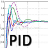# Pidjavadoc

A PID service allows the tracking and transformation of output in relation to iinput.  This is currently used as one of the tracking strategies in the Tracking service.  Input is sent to PID, a "compute" method is sent and appropriate output is sent to a servo.

References

## Examples:

#file : service/Pid.py edit raw
```arduino = Runtime.createAndStart("arduino","Arduino")
arduino.connect("COM5")
# arduino.setSampleRate(30000)

pid = Runtime.start("pid","Pid")

#set Kp, kd, ki kp = gain, how strong it react kd = how fast it react ki= take care of the sum of errors (differences between target and actual value) in the time
pid.setPID("x", 10.0, 0, 1.0)
pid.setMode("x", 1)
#set the range of the "correction"
pid.setOutputRange("x", -5, 5)

# pid.setControllerDirection("x", 0)

#set a starting analog value, which will pilot the MOSFET on the Gate
heaterValue = 512

def onPin(data):
thermistorPin = data
print 'thermistor value is', thermistorPin.value
global heaterValue
global futureHeaterValue
#target of temperature or target value
pid.setSetpoint(150)
#input value
pid.setInput(thermistorPin.value)
pid.compute()
correction = pid.getOutput()
futureHeaterValue = (heaterValue + correction)
if (futureHeaterValue < 1024) and (futureHeaterValue >0):
heaterValue = futureHeaterValue
arduino.analogWrite(4,futureHeaterValue)
print heaterValue
else :
arduino.analogWrite(4,heaterValue)
print heaterValue

```### test [[service/PID.py]]

test [[service/PID.py]]